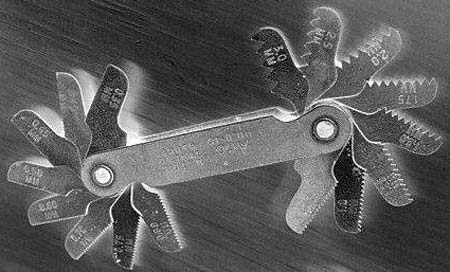X
items
Your shopping cart is empty.
Product Qty Amount
 From: Until:

Archive
[email protected]
/ Categories: Archive, fasteners

# Tensioning using turn-of nut methods

In the previous article on the subject of fasteners, the most commonly used method for the controlled tightening of fasteners was discussed. There are a couple of well-known equations that link tightening torque to fastener tension, and the more reliable of these was discussed. However, there is a great deal of variation of tension for a given torque, even for fasteners of exactly the same design. In terms of the equation itself, differences in the coefficient of friction and the dimensional tolerances of the fasteners in question have significant effects on the torque-tension relationship.

Given that we know the pitch of the bolt, could we not simply arrive at an angle through which we turn the fastener (nut or bolt)? If we assumed the joint (clamped) members to be infinitely stiff, we could simply work back from our knowledge of the axial bolt stiffness and pitch to correlate tightening angle and force.

If the fastener has a stiffness k and pitch p, then for a single complete turn of the fastener head, we assume that the fastener has stretched by a distance p, and that the load is kp. For example, if the fastener stiffness is 50,000 N/mm and the pitch is 1.25 mm, then a single turn should increase the load by 62,500 N, if we assume that the joint is infinitely stiff.

Of course, we have immediately raised the first difficulty - the joint members are certainly not infinitely stiff. The finite stiffness of the clamped members must be taken into account, and if we imagine that the clamped parts are made of rubber, several turns of the fastener described above will develop very little load. Unless the geometry of the joint materials is extremely simple, the calculation of their stiffness is not a trivial matter, and the options for engineers without access to finite element analysis (FEA) software packages are either to turn to physical testing or to calculate the stiffness of the joint members based on assumptions and physical measurements.The calculation of load is made more complicated by the finite stiffness of the clamped joint members, but there are other factors at play too. If the nut is not in contact with the washer when the nut is turned, no tensile load will be developed in the fastener, even if there is a resisting torque (for example from a locking mechanism). That much is obvious, so we may choose not to start measuring the angle of turn until the nut is in contact with the washer.

However, even with the knowledge of accurate fastener and joint stiffness, that is not going to give us the correct relationship between angle of turn and pre-load. The reason is that there is a portion of the tightening event during which the behaviour of the joint is non-linear. Until a certain load is reached, the gradient of the load/angle curve is not constant, and increases until it is equal to the gradient of the linear portion of the curve. If we assume that the load/angle relationship is linear, we stand to have significant differences between the actual fastener load and that desired, especially in cases where the design load is low and the non-linear portion of the chart takes up a significant part of the design load.

Fig. 1 - Knowledge of fastener stiffness, thread pitch and tightening angle won't guarantee accurate bolt pre-load

Written by Wayne Ward

Previous Article E10 - the fuel of the devil?
Next Article MotoGP exhausts